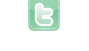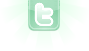## Inaccurate Grid of Squares?

I tried the following code to make a world of 10x10 black squares. But, the squares are not evenly distributed even though in the code seems like they should be. Can anybody provide a fix? Thanks!
```import greenfoot.*;
import java.util.*;

public class Example  extends World
{
private static final int COLUMNS = 10;
private static final int ROWS = 10;
private static final int CELL_SIZE = 10;

public Example()
{
super(COLUMNS, ROWS, CELL_SIZE);
}

public void act()
{
for (int i = 0; i < ROWS; i++)
for (int j = 0; j < COLUMNS; j++)
drawSquare(i, j, Color.BLACK);
}

public void drawSquare(int row, int col, Color color)
{
GreenfootImage square = new GreenfootImage(CELL_SIZE,CELL_SIZE);
square.setColor(color);
square.fillRect(col, row, CELL_SIZE, CELL_SIZE);
getBackground().drawImage(square, col*CELL_SIZE, row*CELL_SIZE);
}

}```
Change line 27 to
`square.fill();`
Or change it it something like this:
`square.fillRect(1, 1, CELL_SIZE-1, CELL_SIZE-1);`
Currently, you are filling a rectangle between (col|row) and (10|10) in the image with the size of 10×10. So the higher col and row get, the smaller the black rectangles will be. You can also move the content of the act method into the constructor.